# DAT Perceptual Ability : DAT Perceptual Ability

## Example Questions

← Previous 1

### Example Question #1 : View Recognition / Top Front End Questions

The pictures that follow are top, front, and end views of solid objects. The views are without perspective. That is, the points in the viewed surface are viewed along parallel lines of vision. The projection looking DOWN on it is shown in the upper left-hand corner (TOP VIEW). The projection looking at the object from the FRONT is shown in the lower left-hand corner (FRONT VIEW). The projection looking at the object from the END is shown in the lower right-hand corner. (END VIEW). These views are ALWAYS in the same positions and are labeled accordingly.

Note that lines that cannot be seen on the surface in some particular view are DOTTED in that view.

In these problems, two views are shown, with four alternatives to complete the set. You are to select the correct one. It is not always the end view that must be selected; sometimes it is the top view or the front view that is missing.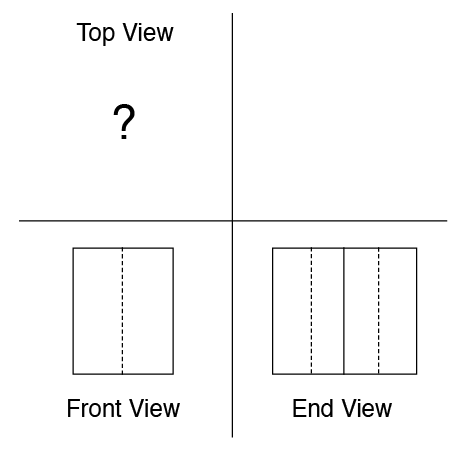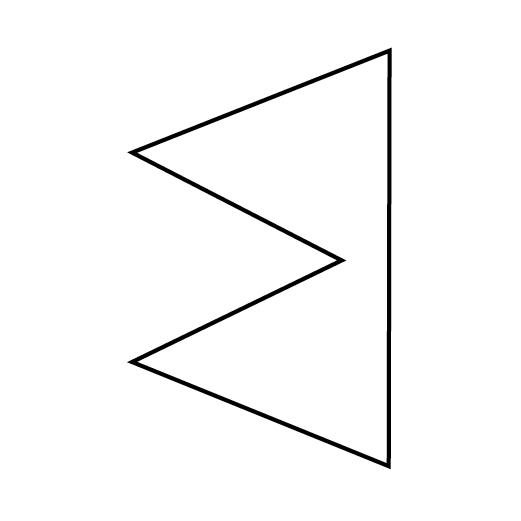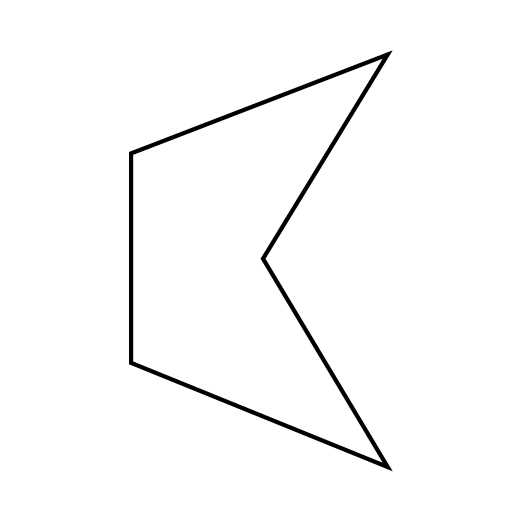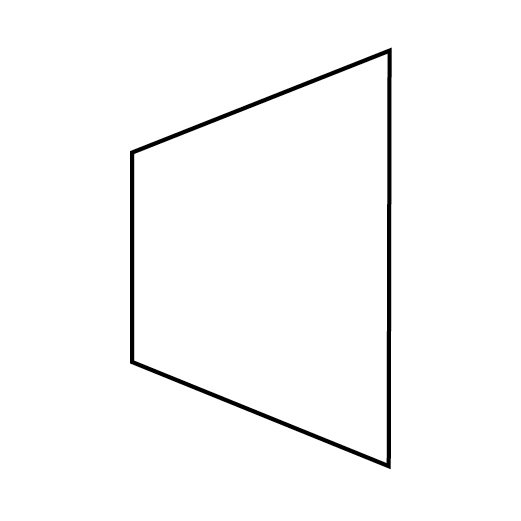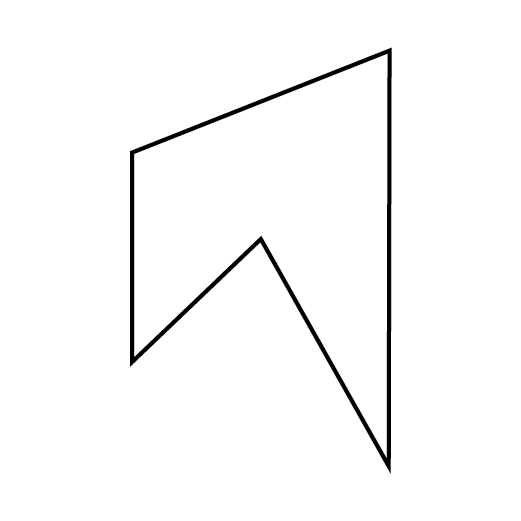Explanation:

To answer this question correctly, you need to understand the difference between solid lines and dashed lines in View Recognition/Top-Front-End questions. Solid lines refer to lines and edges that you can see in a particular view, whereas dashed lines refer to lines and edges that are obscured by something in front of them from that particular view. One tricky part about this question is that when shapes involve angles, the edge on which the vertex of the angle is located is also represented by a line, solid or dotted as necessary.

Considering the front view, we're shown a rectangular face bisected by a dotted line. This tells us that an angle is involved. If a shape built from ninety-degree angles were involved, an even number of lines would be necessary. Since we only see one line, we know that two faces must be coming to a point, either pointing outward from the face we're looking at directly, or inward. It's impossible to tell from just this view. Furthermore, the shape could be an equilateral triangle if these angled edges started at the corners we can see, but alternatively, those edges could extend in space into a prism for a while before angling toward that point. Again, it's impossible to tell from this view alone since these extensions and differences would all occur exactly on the edges we're looking at directly, and thus they wouldn't be shown in this diagram.

Let's now consider what we learn when we combine what we know from the Front View with what we learn from the End View. Now there are three vertical lines. One of them, the center one, is solid, so we are looking head-on at two angled faces. The point could either be coming toward us, away from the shape, or it could extend into the shape. We can't tell. The dotted lines tell us that there are two edges behind the face we're looking at. We don't know if these edges are in line with each other or not, and if they're not, we can't tell which one is closer to us.

Let's look at the answer choices. Since there are so many options as to what these diagrams could represent, let's use process of elimination to figure out which answer choices can't be correct and which one must be correct.This shape can't be correct. While the face at bottom lines up with what we see from the end view (two angled faces meeting in the middle of the shape), the far side of the shape only has one face, not three. We need to see three different faces on the far side of this shape because there are two vertical dotted lines shown in the End View diagram.This shape can't be correct either. While it would involve three faces behind the immediate view if we looked at the right side head-on, there is no angle like the one represented in the Front View diagram. Plus, this diagram is pointed in the wrong direction! Direction is important in Front-End-Side problems.This shape is also incorrect. If we looked at the shape from the bottom, the triangular "indent" would extend much farther to the right of center than is shown in the Front View diagram. In addition, if we looked head-on at the right face of the diagram, we wouldn't see any edges that would match up with the solid vertical line in the End View diagram. That line would have to be dashed like the other two for the End View diagram to be correct.This is the correct answer. If we look at the shape from the front, we see that the triangular indent extends to halfway between the solid vertical line that forms the left face and the points of the rightmost part of the shape. The vertical line would be represented by the left vertical line in the Front View, and the right vertical line would be represented by a line extending downward from the "points" of the right side of the shape. Since these points are in line with one another, only one line would be required for the right side in the Front View diagram. Seen from the right for the End View, the solid vertical line in the middle represents the middle of the indented angle, and the two vertical lines represent the edges where on the left part of the top view, the sides angle out from the vertical line.

### Example Question #1 : View Recognition / Top Front End Questions

The pictures that follow are top, front, and end views of solid objects. The views are without perspective. That is, the points in the viewed surface are viewed along parallel lines of vision. The projection looking DOWN on it is shown in the upper left-hand corner (TOP VIEW). The projection looking at the object from the FRONT is shown in the lower left-hand corner (FRONT VIEW). The projection looking at the object from the END is shown in the lower right-hand corner. (END VIEW). These views are ALWAYS in the same positions and are labeled accordingly.

Note that lines that cannot be seen on the surface in some particular view are DOTTED in that view.

In these problems, two views are shown, with four alternatives to complete the set. You are to select the correct one. It is not always the end view that must be selected; sometimes it is the top view or the front view that is missing.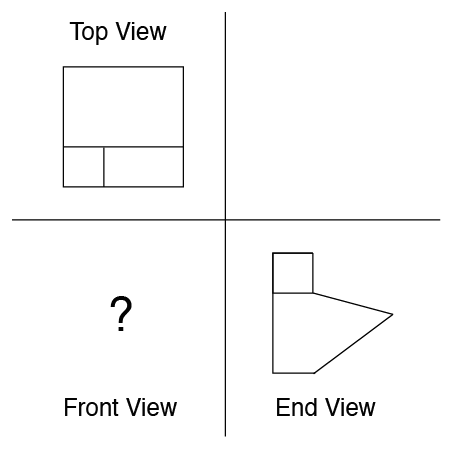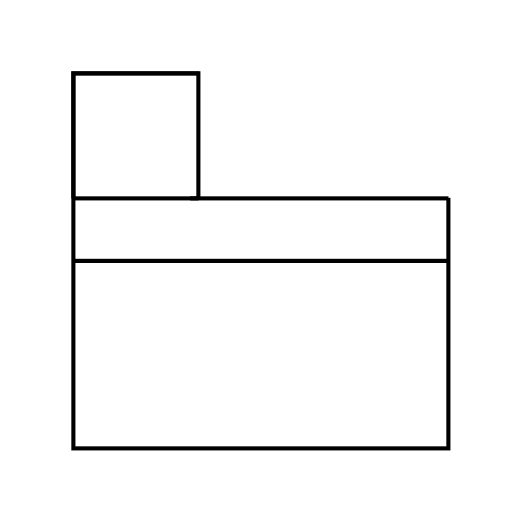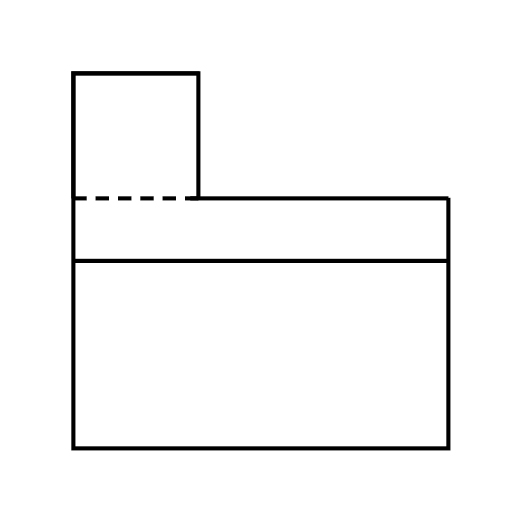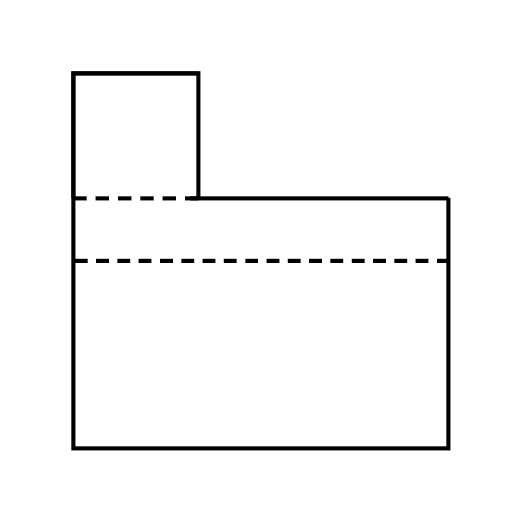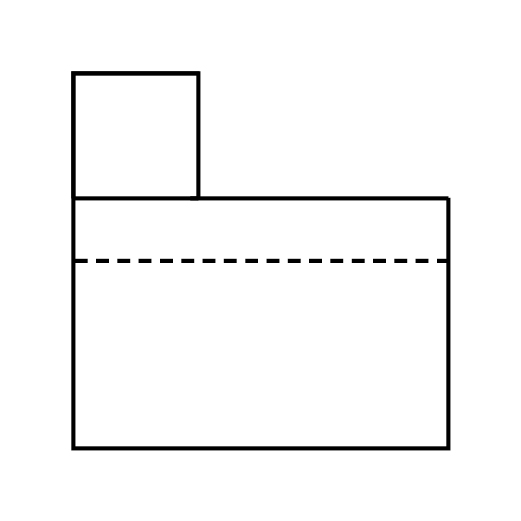Explanation:

To answer this question correctly, you need to understand the difference between solid lines and dashed lines on the DAT Perceptual Ability section. In this case, we're looking for the front view. The top view of this shape shows us that it is divided into three sections. Considering just this view, this could mean a variety of different things: the shape might be cube-like and each section might be a different step, for example, but we'd have no information about how the heights of each "step" would compare. In addition, the shape might not be a cube; angles might be involved. We can't tell solely from looking at the top view, though. The end view provides us with more information that allows us to narrow down our interpretation of the top view. Here, we see that the shape, when viewed from the end, involves an angle, and that there is a solid line at the bottom of the cube above the angular piece. This tells us that the cube is not connected to the shape that includes the angle. The cube's face isn't connected to the end face of the angled piece; it is separate. This tells us that the cube is farther away than the angled end face facing us directly in the end view. If one of its faces were connected to the angled end face, the diagram would not include a line separating the "cube" part of the shape from the angled part of the shape.

Now let's consider what the front of the shape has to look like. Notice that the "cube" part of the shape is on the left in all of the answer choices. This is important, as it tells us that we are looking at the flat, rectangular side of the larger part of the shape head-on, not at the angled sides. If we were looking at the angled sides, the "cube" part would have to be on the right side of the front-view diagram, and this isn't an option in any of the answer choices.

So, we know that the line forming the vertex of the angle shown in the End View diagram is behind the face of the shape we're directly looking at in the Front View. This tells us that this line must be dotted, not solid. Figuring this out allows us to ignore the following two answer choices because they use solid lines for their bottom-most shape-crossing line:This leaves us with two remaining answer choices, each of which correctly uses a dotted line:The only difference between these two answer choices is that the one on the left uses a dashed line to divide the "cube" shape from the rest of the shape, and the one on the right uses a solid line for this division. Which should it be? Consider how this "cube" shape will appear when we look at the shape head-on with the face shown as the bottom line of the Top View appearing first. Is the "cube" part of the shape in line with this face, or is it located further back in space? If the former is true, we need to use a dotter line to show that the cube shape does not continue for the entire volume of the shape, but that there is negative space located behind it. If the latter is true, we need to use a solid line to show that the negative space is in front of the cube. Looking at the Top View diagram, we can see that one face of the cube will be even with the face of the larger part of the shape at which we are looking in the Front View. This means that the negative space appears behind the cube, not in front of it, making the correct answer the shape that uses the dotted line:### Example Question #1 : Aperture / Keyhole Questions

A three-dimensional object is shown in the question prompt, and outlines of five apertures or openings are shown as the answer choices. First, you imagine how the object looks from all directions (rather than form a single direction as shown). Then, pick from the five apertures outlined the opening through which the object could pass directly if the proper side were inserted first.

Here are the rules:

1. Prior to passing through the aperture, the irregular solid object may be turned in any direction. It may be started through the aperture on a side not shown.

2. Once the object is started through the aperture, it may not be twisted or turned. It must pass completely through the opening. The opening is always the exact shape of the appropriate external outline of the object.

3. Both objects are drawn to the same scale. Thus, it is possible for an opening to be the correct shape but too small for the object; however, in all cases, differences are large enough to judge by eye.

4. There are no irregularities in any hidden portion of the object; however, if the figure has symmetric indentations, the hidden portion is symmetric with the part shown.

5. For each object there is only one correct answer.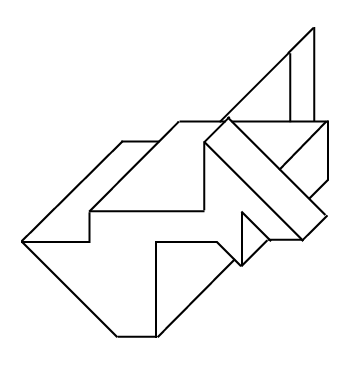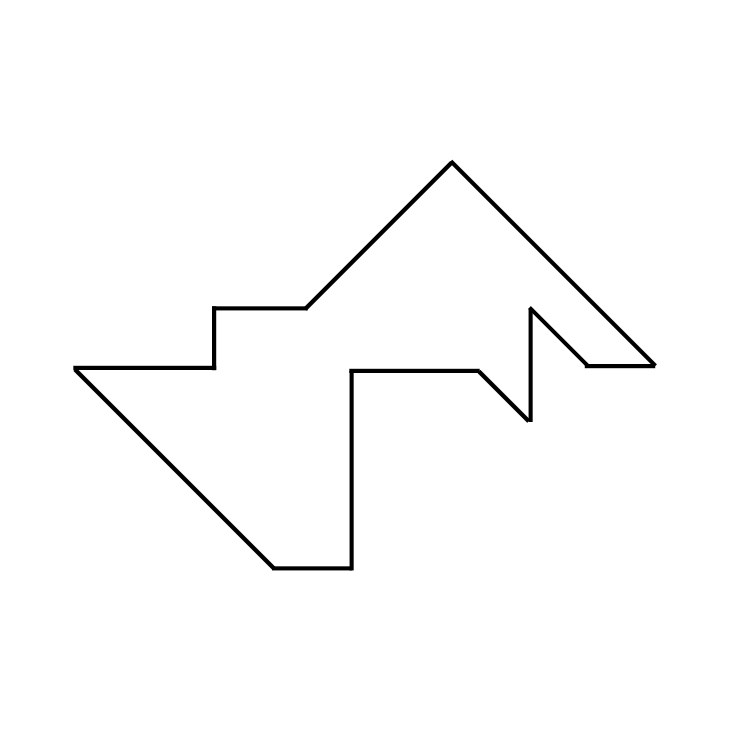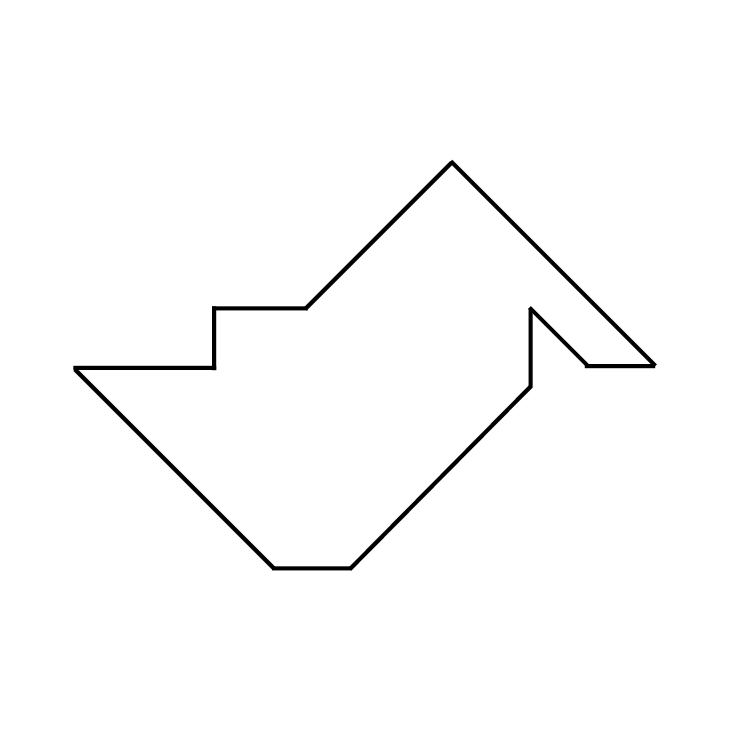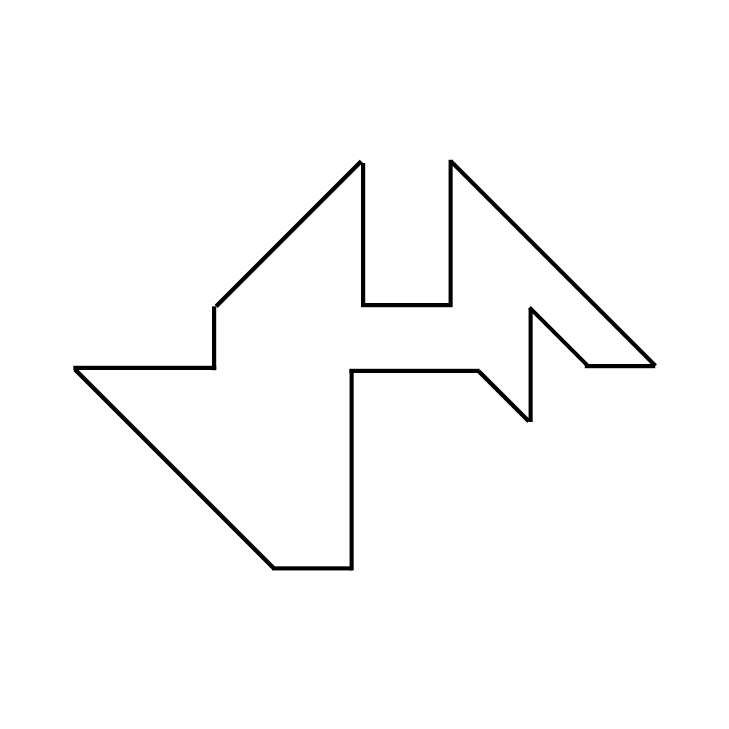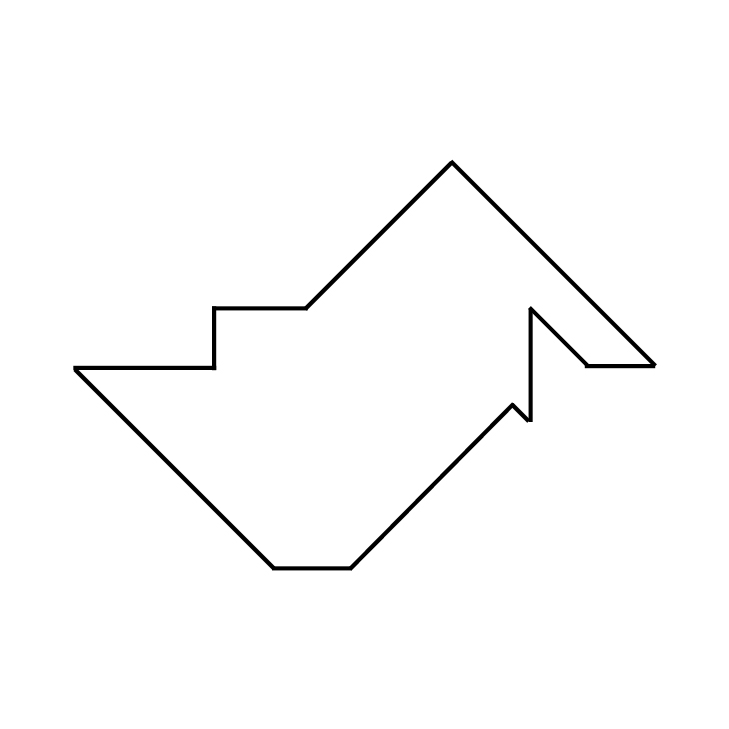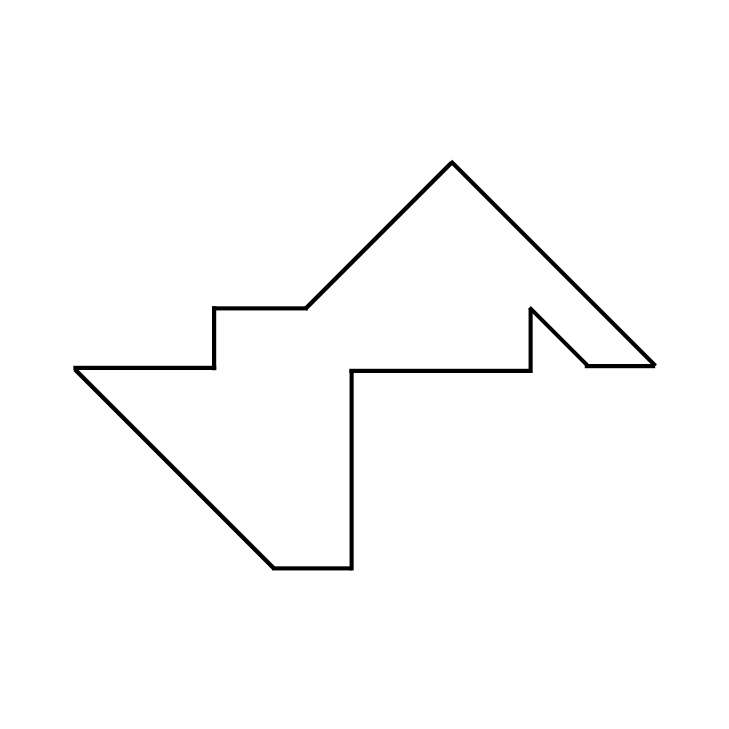Explanation:

This is the correct keyhole/aperture shape:If the three-dimensional shape were positioned so that the front-most face were parallel with the keyhole, it would be able to fit through. Note how the triangular piece that juts up above the mostly-rectangular prism farthest away from the presented three-dimensional view would require the left sloped side of the triangular portion near the top of the keyhole, and the triangular piece sticking up from the front of the presented three-dimensional view would require the right sloped side of the triangle in the keyhole. The bottom right corner of the mostly-rectangular prism comes to a point, which is accommodated by a corresponding point in the keyhole. The right angle where the mostly-rectangular prism meets the left part of the shown three-dimensional shape is also represented in the keyhole.The shape showns above are not correct because neither shows the right angle where the rectangular prism with a pointed bottom-right corner meets the left part of the shape. In addition, the shape on the right does not provide space for the pointed corner near the bottom-right of the presented view.This shape is not correct because the two triangular pieces that jut above the mostly-rectangular prism are shown with a gap in between them. By looking at the presented three-dimensional shape, you can see that they do not have this large of a gap in between their spacing on the parallel sides of the rectangular prism.This shape is not correct because it does not include space for the pointed corner of the shape similar to a rectangular prism. This is its bottom-right corner in the presented view.

### Example Question #1 : Aperture / Keyhole Questions

A three-dimensional object is shown in the question prompt, and outlines of five apertures or openings are shown as the answer choices. First, you imagine how the object looks from all directions (rather than form a single direction as shown). Then, pick from the five apertures outlined the opening through which the object could pass directly if the proper side were inserted first.

Here are the rules:

1. Prior to passing through the aperture, the irregular solid object may be turned in any direction. It may be started through the aperture on a side not shown.

2. Once the object is started through the aperture, it may not be twisted or turned. It must pass completely through the opening. The opening is always the exact shape of the appropriate external outline of the object.

3. Both objects are drawn to the same scale. Thus, it is possible for an opening to be the correct shape but too small for the object; however, in all cases, differences are large enough to judge by eye.

4. There are no irregularities in any hidden portion of the object; however, if the figure has symmetric indentations, the hidden portion is symmetric with the part shown.

5. For each object there is only one correct answer.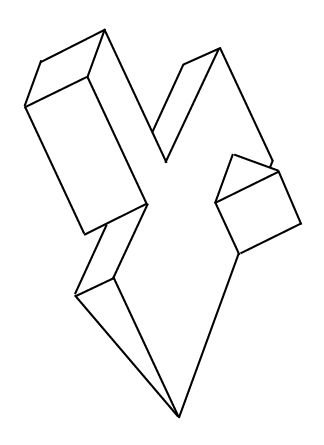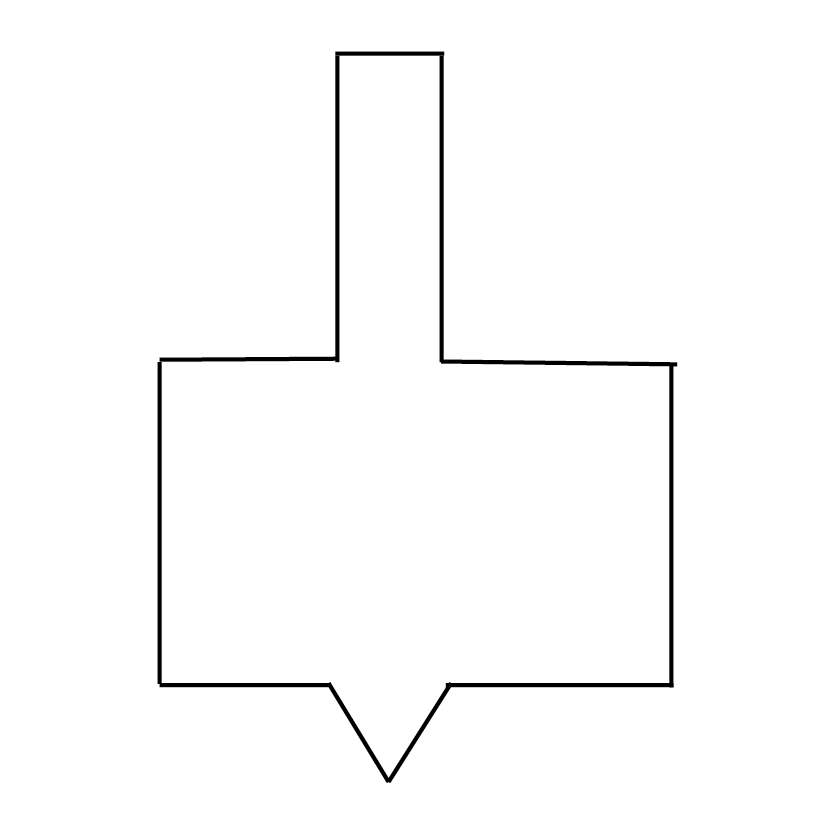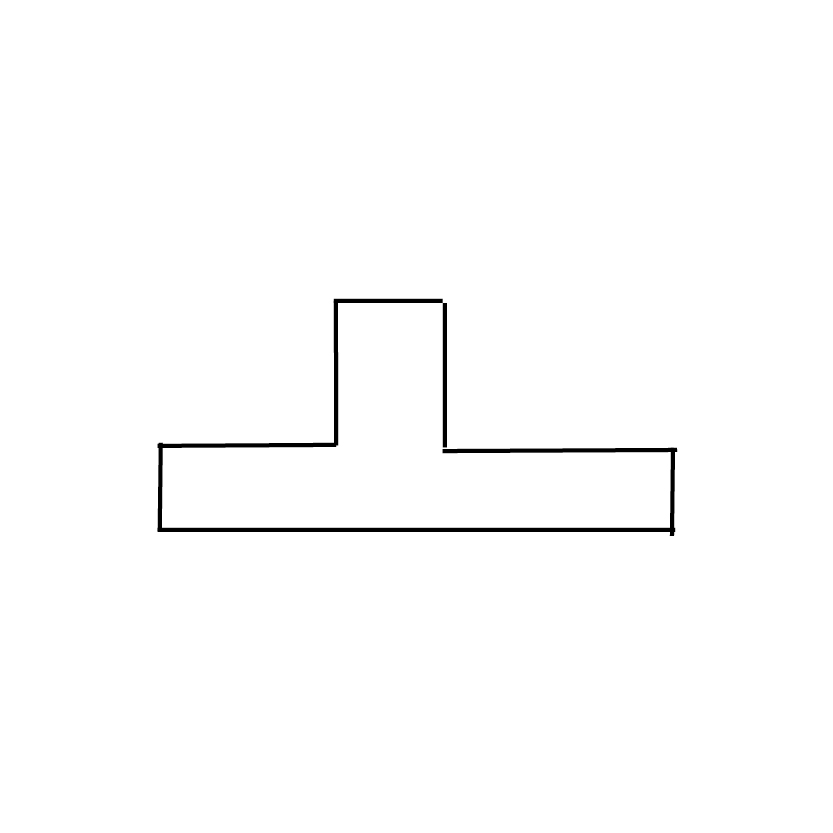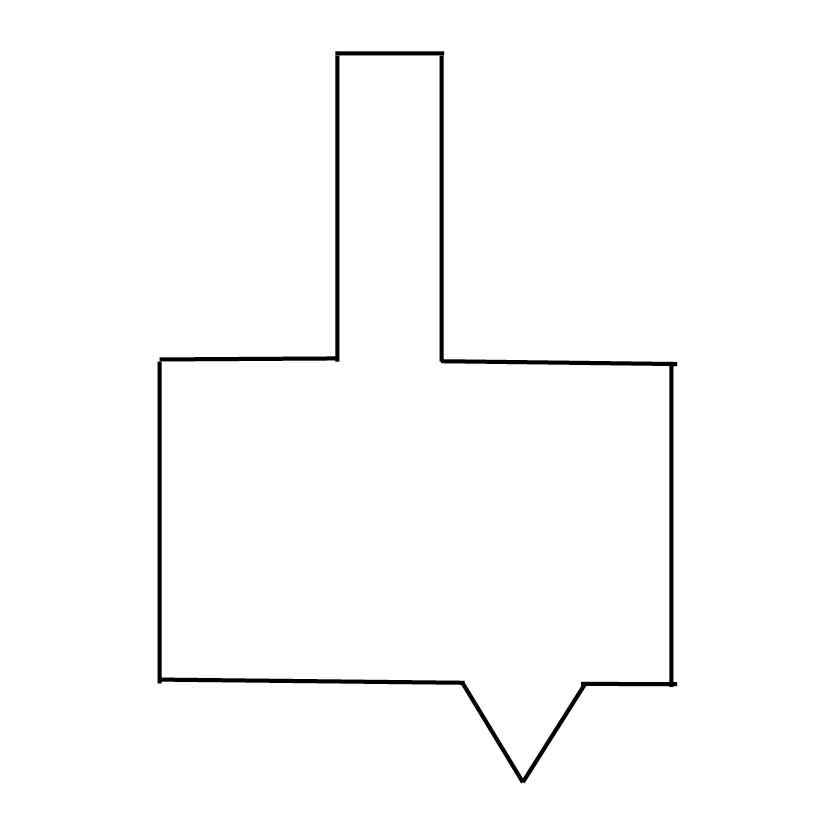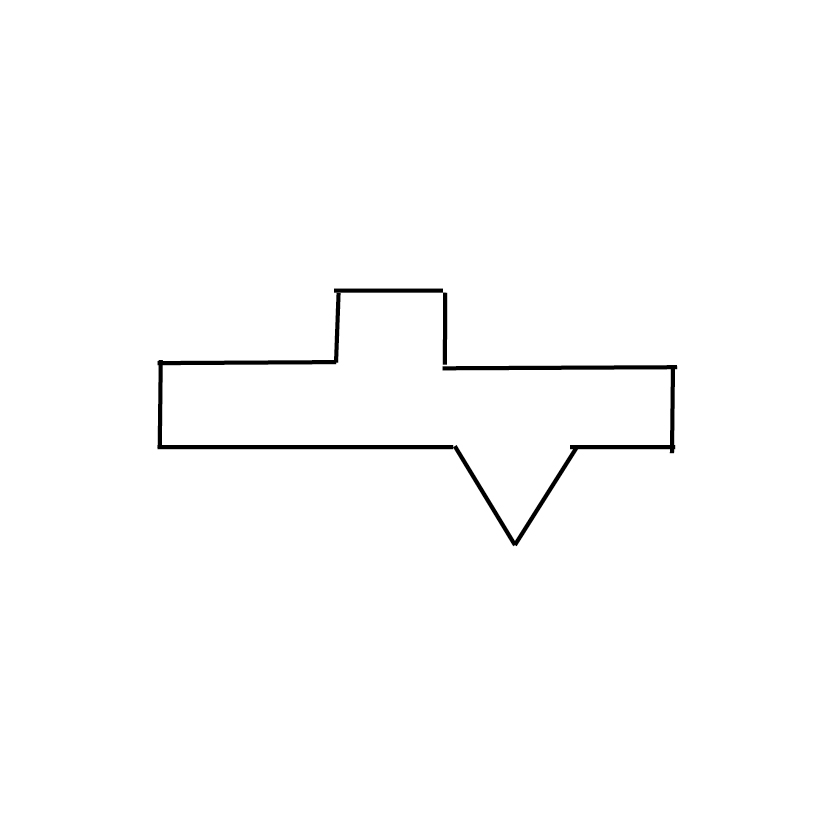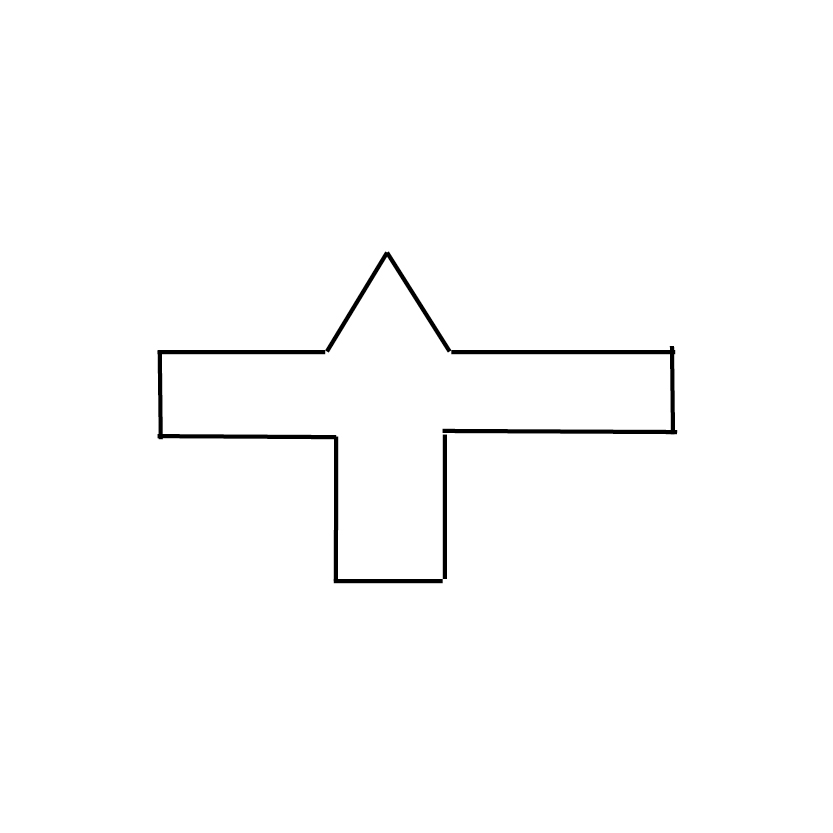Explanation:

This question deals with a shape composed of three parts. On the left of the presented three-dimensional view is a long rectangular prism; this merges on one face into a triangular prism, and on the right side of the presented view, we see that a smaller triangular prism.

The answer choices can be divided into two categories: those that involve the largest face of the large triangular prism in the middle of the shape . . .. . . and those that involve the smaller rectangular face of the triangular prism shown at the upper-left of the presented view:Consider how the smaller triangular prism relates to the largest face of the larger triangular prism. If you looked at the largest face of the larger triangular prism, would any part of the smaller triangular prism stick out of the outline? It would not, since this smaller prism is resting on that large face and doesn't stick out past its edges. We can thus ignore the two answer choices that use shapes showing the largest face of the large triangular prism, because both of these answer choices incorrectly show a triangular indent in the edge.

We now need to consider how the shape would look if we viewed it head-on from the upper-left face of the larger triangular prism. Would the rectangular prism and the smaller triangular prism both stick out from the overall rectangular outline of this face? Yes, they would. So, the middle shape shown in the three above is incorrect because it only shows an indentation for the prism and not for the triangular prism. In deciding between the remaining two shapes, remember: it doesn't matter whether the triangle or the rectangular prism is shown on the top or on the bottom of the longer rectangle. The three-dimensional shape could be rotated to make either admissible. What, then, differentiates these answers? In the shape shown on the left (or top) of the three above, the rectangular indentation is shorter than the one included in the keyhole/aperture shown on the right. In addition, the rectangular indentation and the triangular indentation are staggered, whereas in the keyhole/aperture on the far right (or bottom), they are immediately above one another.

This gives us all the information we need to answer this question correctly. You can see from the three-dimensional shape that the rectangular prism and the smaller triangular prism do not line up with one another in this way. In addition, the shorter indent for the rectangular prism makes sense, because part of its height is shared with the height of the small, upper-right face of the large triangular prism. The correct answer is the following keyhole/aperture:### Example Question #1 : Angle Discrimination / Angle Ranking Questions

For this question, examine the for INTERIOR angles and rank each in terms of degrees from SMALL TO LARGE. Choose the answer choice that has the correct ranking.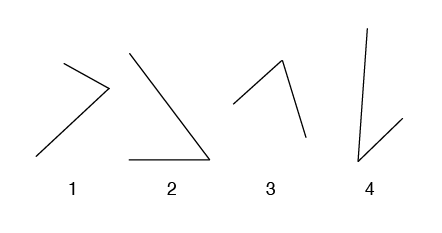4 - 2 - 1 - 3

2 - 4 - 3 - 1

2 - 4 - 1 - 3

4 - 2 - 3 - 1

4 - 2 - 3 - 1

Explanation: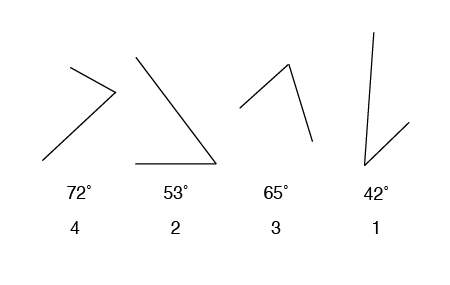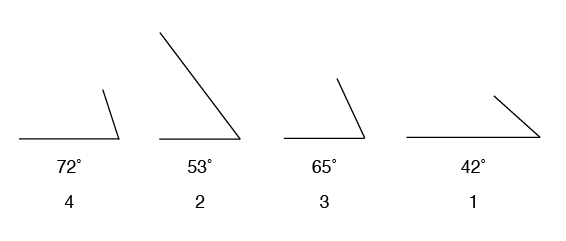### Example Question #1 : Angle Discrimination / Angle Ranking Questions

For this question, examine the for INTERIOR angles and rank each in terms of degrees from SMALL TO LARGE. Choose the answer choice that has the correct ranking.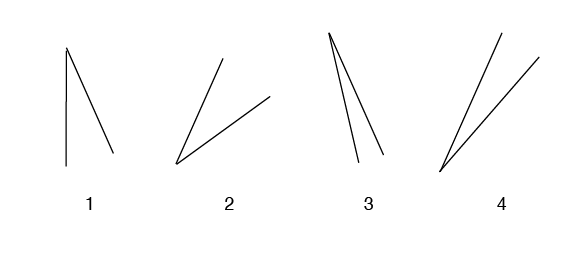3 - 4 - 1 - 2

3 - 4 - 2 - 1

4 - 3 - 2 - 1

4 - 3 - 1 - 2

3 - 4 - 1 - 2

Explanation: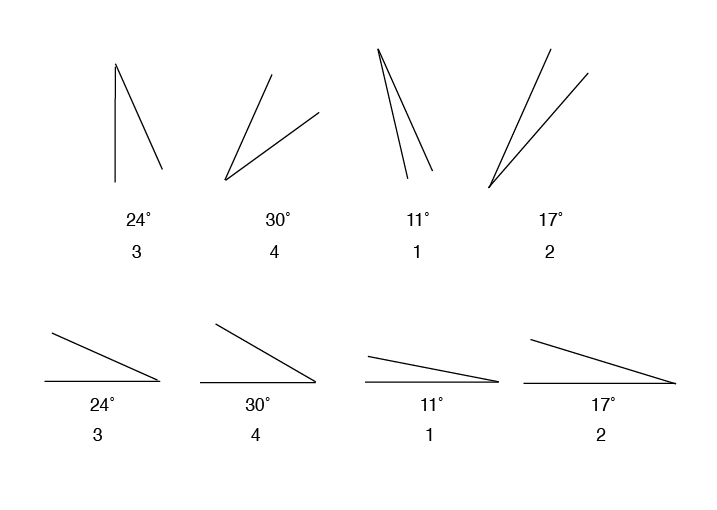### Example Question #1 : Paper Folding / Hole Punching Questions

A flat square of paper is folded one or more times. The broken lines indicate the original position of the paper. The solid lines indicate the position of the folded paper. The paper is never turned or twisted. The folded paper always remains within the edges of the original square. There may be from one to three folds in each item. After the last fold, a hole is punched in the paper. Your task is to mentally unfold the paper and determine the position of the holes on the original square. Choose the pattern of black circles that indicates the position of the holes on the original square. There is only one correct pattern for each item.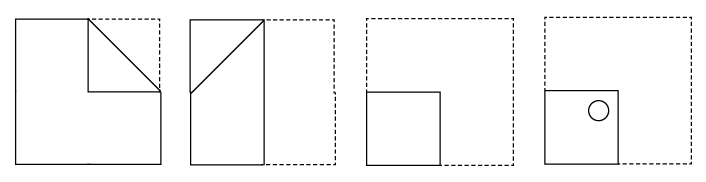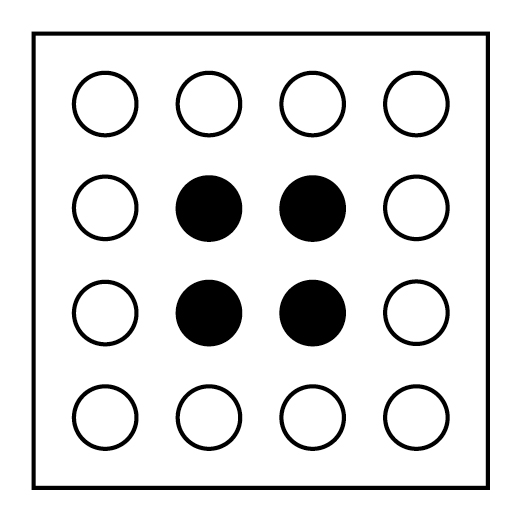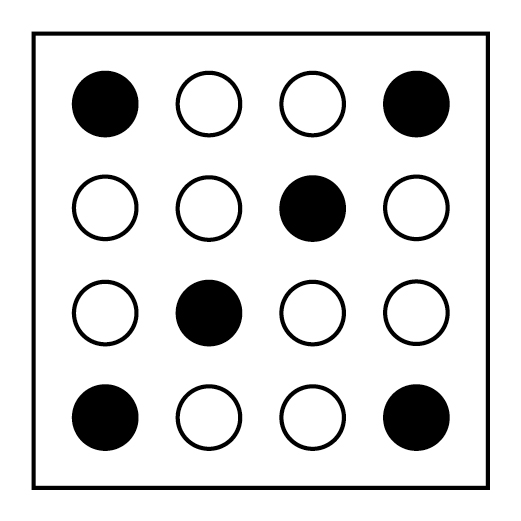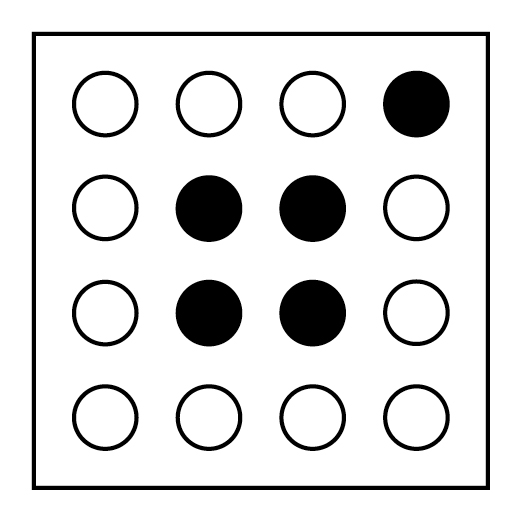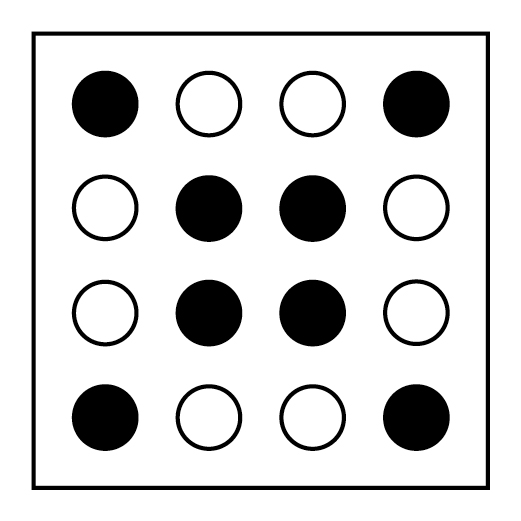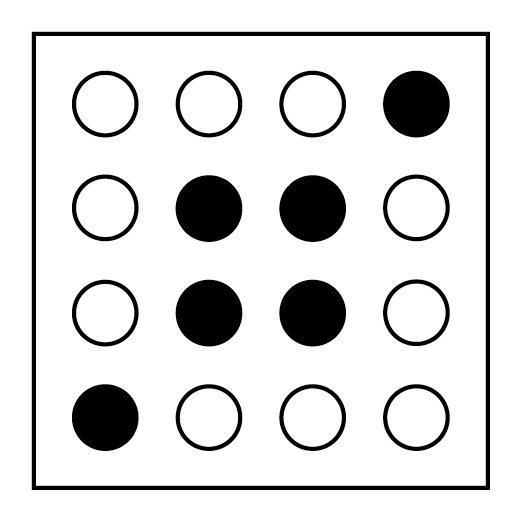Explanation: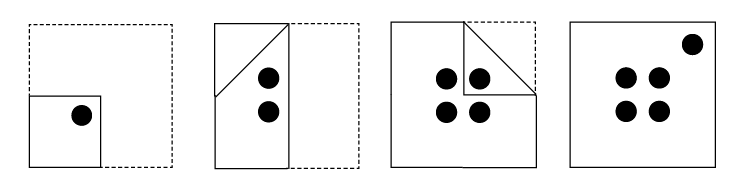### Example Question #1 : Dat Perceptual Ability

A flat square of paper is folded one or more times. The broken lines indicate the original position of the paper. The solid lines indicate the position of the folded paper. The paper is never turned or twisted. The folded paper always remains within the edges of the original square. There may be from one to three folds in each item. After the last fold, a hole is punched in the paper. Your task is to mentally unfold the paper and determine the position of the holes on the original square. Choose the pattern of black circles that indicates the position of the holes on the original square. There is only one correct pattern for each item.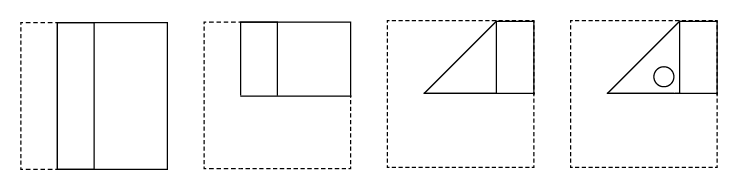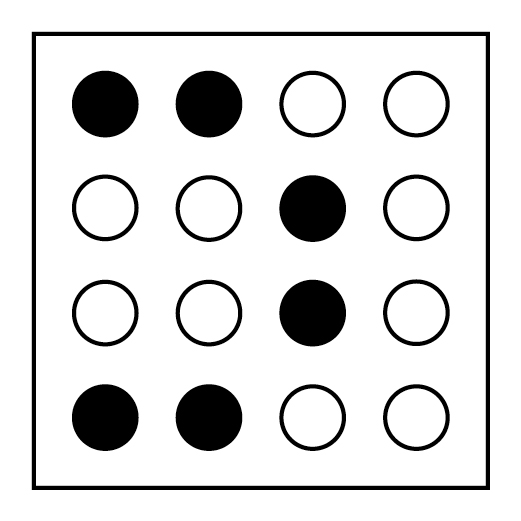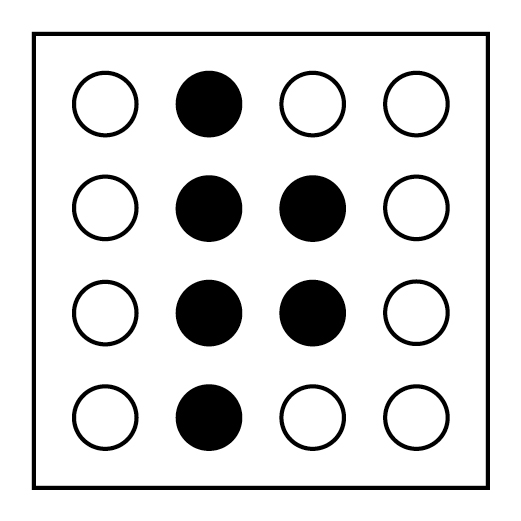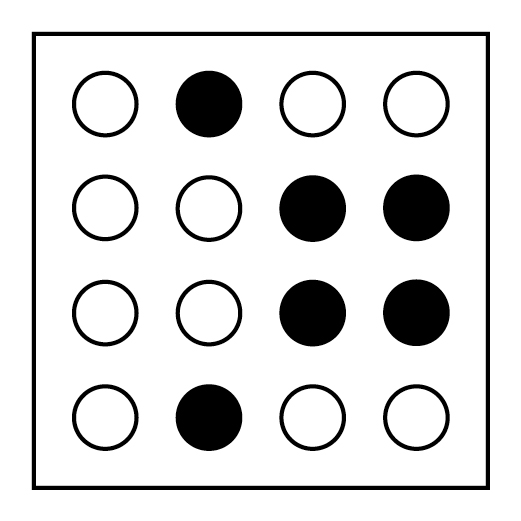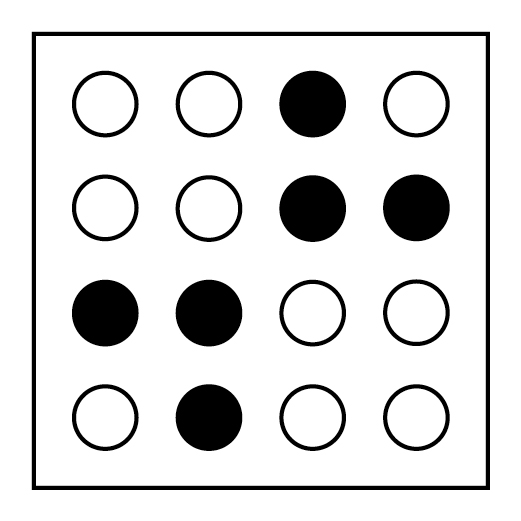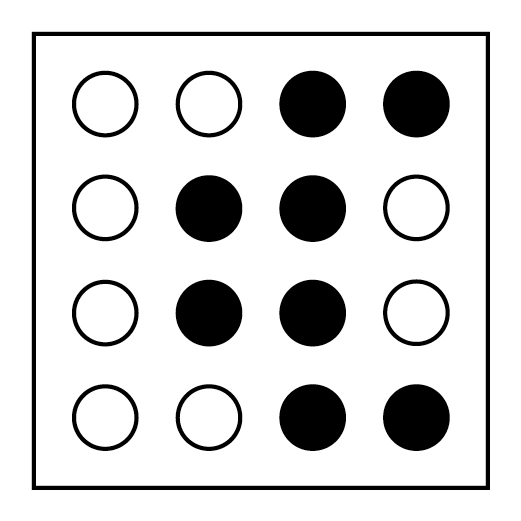Explanation: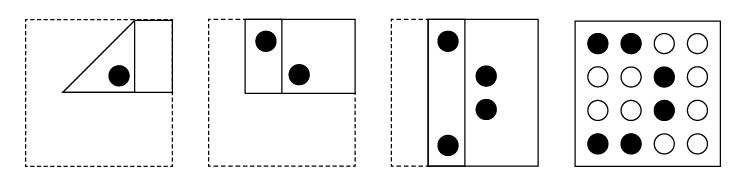### Example Question #1 : Cube Counting Questions

Consider the following figure, which has been made by cementing together cubes of the same size. After being cemented, each group was painted on all sides except for the bottom on which it is resting. Not all cubes may be visible in the figure, but the only hidden cubes are those required to support other cubes.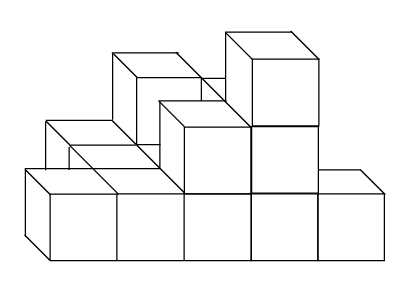In the image shown above, how many of the cubes have four of their sides painted?

1 cube

5 cubes

3 cubes

4 cubes

2 cubes

4 cubes

Explanation: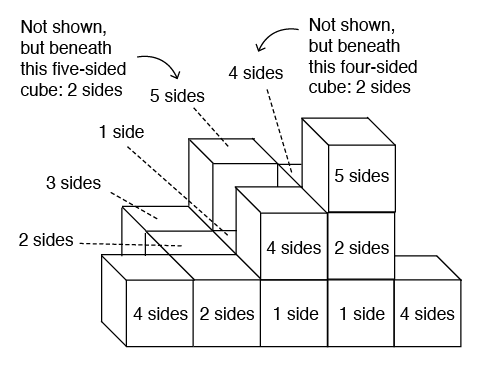### Example Question #2 : Cube Counting Questions

Consider the following figure, which has been made by cementing together cubes of the same size. After being cemented, each group was painted on all sides except for the bottom on which it is resting. Not all cubes may be visible in the figure, but the only hidden cubes are those required to support other cubes.In the figure shown above, how many cubes have only one of their sides painted?

3 cubes

5 cubes

1 cube

4 cubes

2 cubes